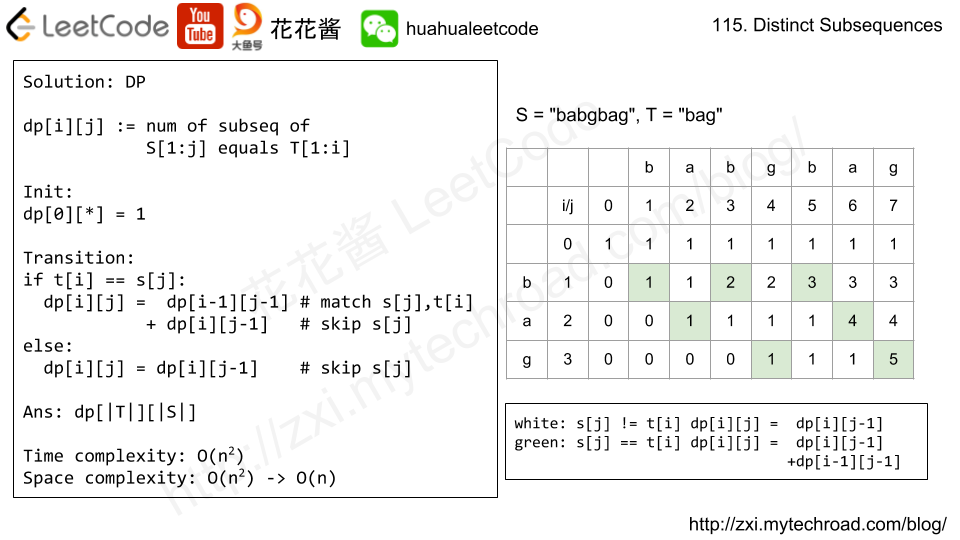# Posts tagged as “counting”

You are given a map of a server center, represented as a m * n integer matrix grid, where 1 means that on that cell there is a server and 0 means that it is no server. Two servers are said to communicate if they are on the same row or on the same column.

Return the number of servers that communicate with any other server.

Example 1:

Input: grid = [[1,0],[0,1]]
Output: 0
Explanation: No servers can communicate with others.

Example 2:

Input: grid = [[1,0],[1,1]]
Output: 3
Explanation: All three servers can communicate with at least one other server.


Example 3:

Input: grid = [[1,1,0,0],[0,0,1,0],[0,0,1,0],[0,0,0,1]]
Output: 4
Explanation: The two servers in the first row can communicate with each other. The two servers in the third column can communicate with each other. The server at right bottom corner can't communicate with any other server.


Constraints:

• m == grid.length
• n == grid[i].length
• 1 <= m <= 250
• 1 <= n <= 250
• grid[i][j] == 0 or 1

## Solution: Counting

Two passes:
First pass, count number of computers for each row and each column.
Second pass, count grid[i][j] if rows[i] or cols[j] has more than 1 computer.

Time complexity: O(m*n)
Space complexity: O(m + n)

## C++

Given n, how many structurally unique BST’s (binary search trees) that store values 1 … n?

Example:

Input: 3
Output: 5
Explanation:
Given n = 3, there are a total of 5 unique BST's:

1         3     3      2      1
\       /     /      / \      \
3     2     1      1   3      2
/     /       \                 \
2     1         2                 3

## Solution: DP

dp[i] = sum(dp[j] * dp[i – j – 1]) (0 <= j < i )

root: 1 node
left child: j nodes
right child i – j – 1 nodes

try all possible partitions

ans = dp[n]

Time complexity: O(n^2)
Space complexity: O(n)

## C++

Given an array of integers A, find the number of triples of indices (i, j, k) such that:

• 0 <= i < A.length
• 0 <= j < A.length
• 0 <= k < A.length
• A[i] & A[j] & A[k] == 0, where & represents the bitwise-AND operator.

Example 1:

Input: [2,1,3]
Output: 12
Explanation: We could choose the following i, j, k triples:
(i=0, j=0, k=1) : 2 & 2 & 1
(i=0, j=1, k=0) : 2 & 1 & 2
(i=0, j=1, k=1) : 2 & 1 & 1
(i=0, j=1, k=2) : 2 & 1 & 3
(i=0, j=2, k=1) : 2 & 3 & 1
(i=1, j=0, k=0) : 1 & 2 & 2
(i=1, j=0, k=1) : 1 & 2 & 1
(i=1, j=0, k=2) : 1 & 2 & 3
(i=1, j=1, k=0) : 1 & 1 & 2
(i=1, j=2, k=0) : 1 & 3 & 2
(i=2, j=0, k=1) : 3 & 2 & 1
(i=2, j=1, k=0) : 3 & 1 & 2


Note:

1. 1 <= A.length <= 1000
2. 0 <= A[i] < 2^16

## Solution: Counting

Time complexity: O(n^2 + n * max(A))
Space complexity: O(max(A))

# Problem

Given a string S and a string T, count the number of distinct subsequences of S which equals T.

A subsequence of a string is a new string which is formed from the original string by deleting some (can be none) of the characters without disturbing the relative positions of the remaining characters. (ie, "ACE" is a subsequence of "ABCDE" while "AEC" is not).

Example 1:

Input: S = "rabbbit", T = "rabbit"
Output: 3
Explanation:  As shown below, there are 3 ways you can generate "rabbit" from S. (The caret symbol ^ means the chosen letters)
rabbbit
^^^^ ^^
rabbbit
^^ ^^^^
rabbbit
^^^ ^^^

Example 2:

Input: S = "babgbag", T = "bag"
Output: 5
Explanation:  As shown below, there are 5 ways you can generate "bag" from S. (The caret symbol ^ means the chosen letters)
babgbag
^^ ^
babgbag
^^ ^
babgbag
^ ^^
babgbag
^ ^^
babgbag
^^^

# Solution: DPTime complexity: O(|s| * |t|)

Space complexity: O(|s| * |t|)

C++

# Problem

There is an m by n grid with a ball. Given the start coordinate (i,j) of the ball, you can move the ball to adjacent cell or cross the grid boundary in four directions (up, down, left, right). However, you can at most move N times. Find out the number of paths to move the ball out of grid boundary. The answer may be very large, return it after mod 109 + 7.

Example 1:

Input:m = 2, n = 2, N = 2, i = 0, j = 0
Output: 6
Explanation:Example 2:

Input:m = 1, n = 3, N = 3, i = 0, j = 1
Output: 12
Explanation:Note:

1. Once you move the ball out of boundary, you cannot move it back.
2. The length and height of the grid is in range [1,50].
3. N is in range [0,50].

# Solution

Time complexity: O(m*n + N * m * n)

Space complexity: O(N*m*n) -> O(m*n)

C++

Recursion with memorization

no loops

# Related Problems

Mission News Theme by Compete Themes.